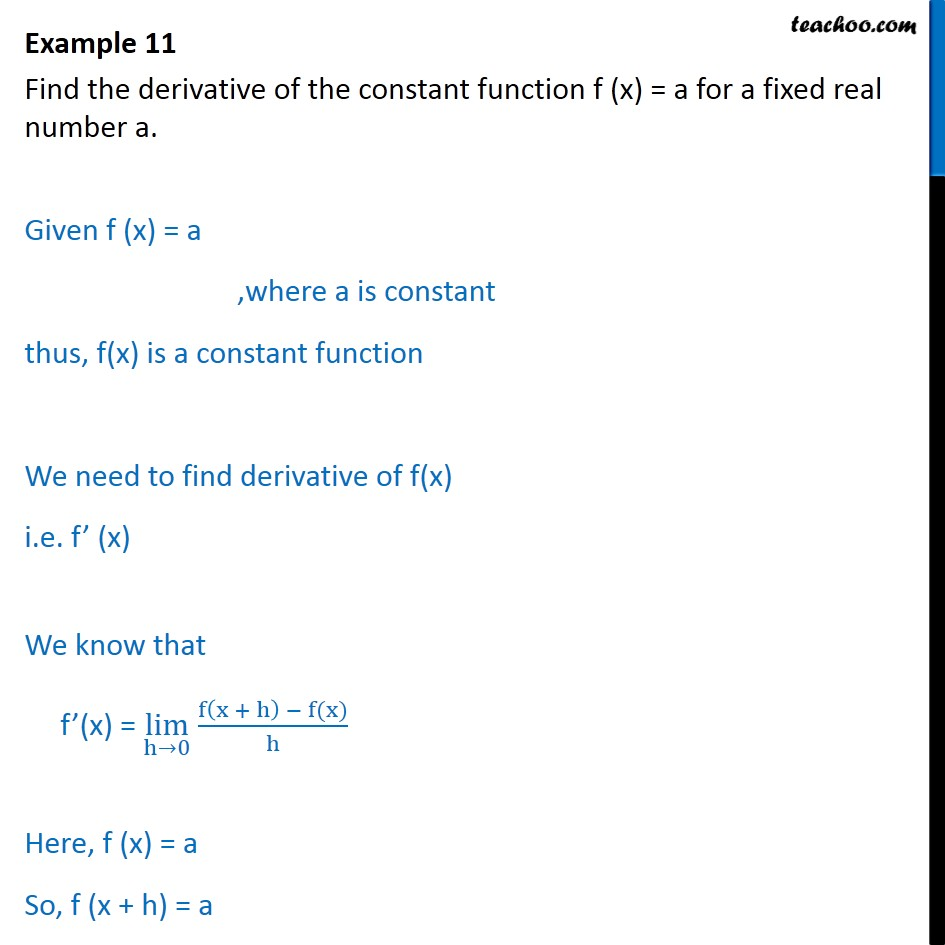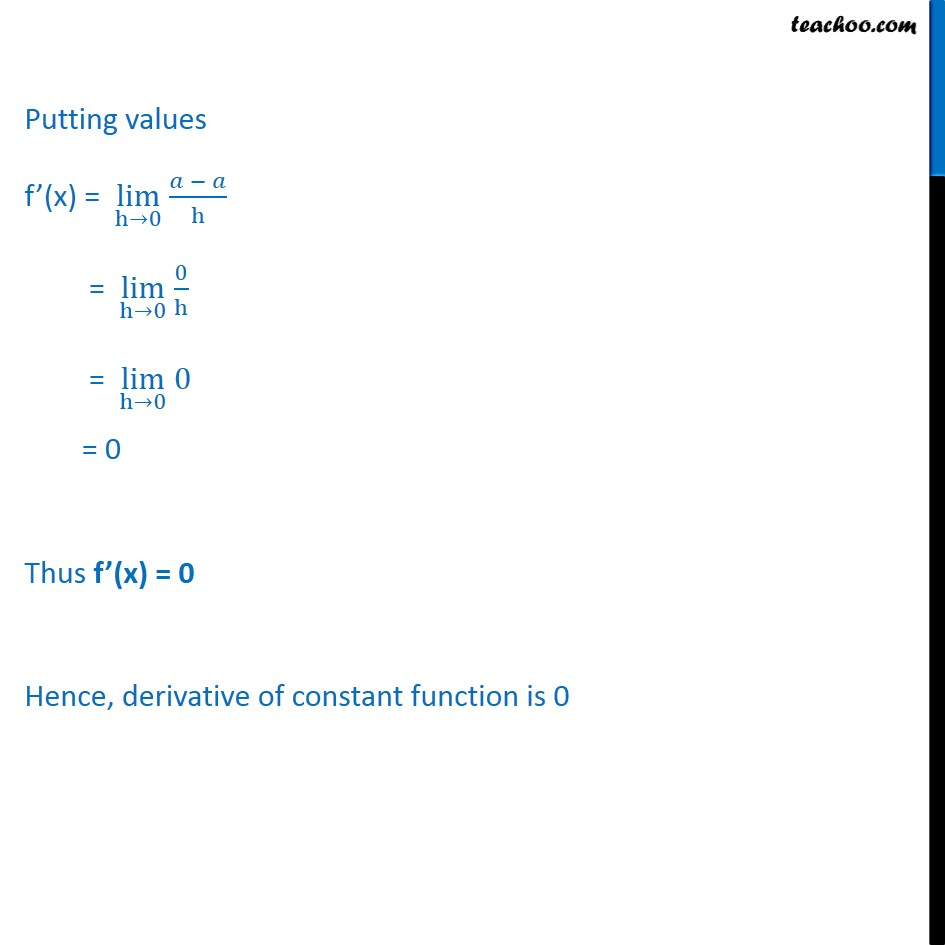Examples (Term 1 and Term 2)

Chapter 13 Class 11 Limits and Derivatives (Term 1 and Term 2)
Serial order wise### Transcript

Example 11 Find the derivative of the constant function f (x) = a for a fixed real number a. Given f (x) = a ,where a is constant thus, f(x) is a constant function We need to find derivative of f(x) i.e. f (x) We know that f (x) = lim h 0 f x + h f(x) h Here, f (x) = a So, f (x + h) = a Putting values f (x) = lim h 0 h = lim h 0 0 h = lim h 0 0 = 0 Thus f (x) = 0 Hence, derivative of constant function is 0